# Graphing with Functions: Homework Help Chapter Exam

Exam Instructions:

Choose your answers to the questions and click 'Next' to see the next set of questions. You can skip questions if you would like and come back to them later with the yellow "Go To First Skipped Question" button. When you have completed the practice exam, a green submit button will appear. Click it to see your results. Good luck!

### Page 1

#### Question 3 3. How many functions y=f(x) are needed to represent the graph below?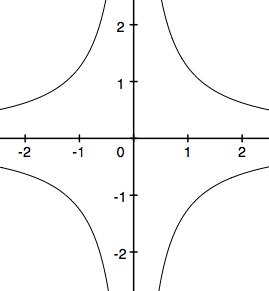#### Question 5 5. The implicit function x^2=y^2 is graphed below. Which functions give the same graph?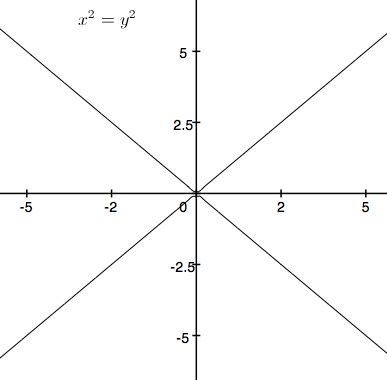### Page 2

#### Question 7 7. What is the slope of the line tangent to the curve given by the equation y = (x-1)²+2 at the point (1,2)?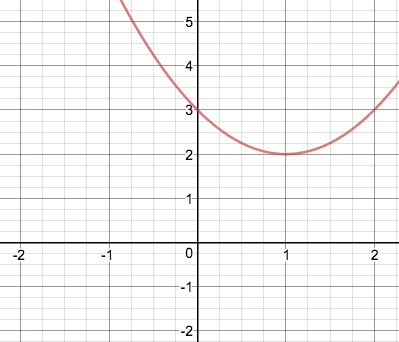### Page 5

#### Question 25 25. What are the asymptotes for this equation?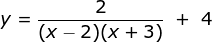### Page 6

#### Question 28 28. If the following graph is an implicit function, then how many real functions would it take to graph it?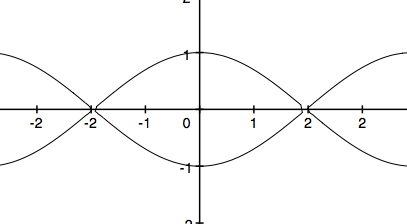#### Graphing with Functions: Homework Help Chapter Exam Instructions

Choose your answers to the questions and click 'Next' to see the next set of questions. You can skip questions if you would like and come back to them later with the yellow "Go To First Skipped Question" button. When you have completed the practice exam, a green submit button will appear. Click it to see your results. Good luck!

Support